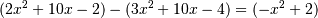# numpy.polysub¶

`numpy.``polysub`(a1, a2)[source]

Difference (subtraction) of two polynomials.

Given two polynomials a1 and a2, returns `a1 - a2`. a1 and a2 can be either array_like sequences of the polynomials’ coefficients (including coefficients equal to zero), or `poly1d` objects.

Parameters: a1, a2 : array_like or poly1d Minuend and subtrahend polynomials, respectively. out : ndarray or poly1d Array or `poly1d` object of the difference polynomial’s coefficients.

Examples```>>> np.polysub([2, 10, -2], [3, 10, -4])
array([-1,  0,  2])
```

numpy.polymul

#### Next topic

numpy.RankWarning